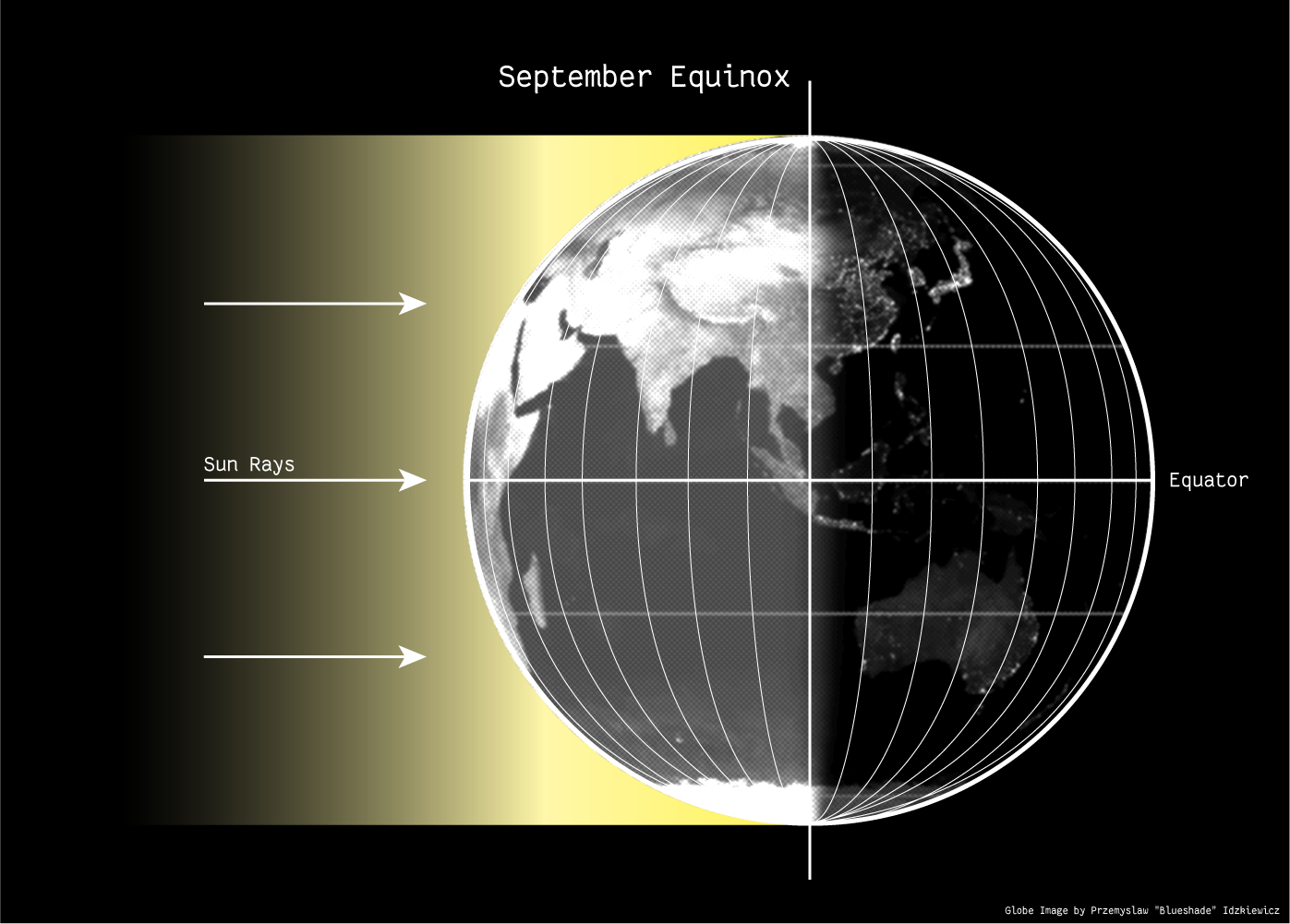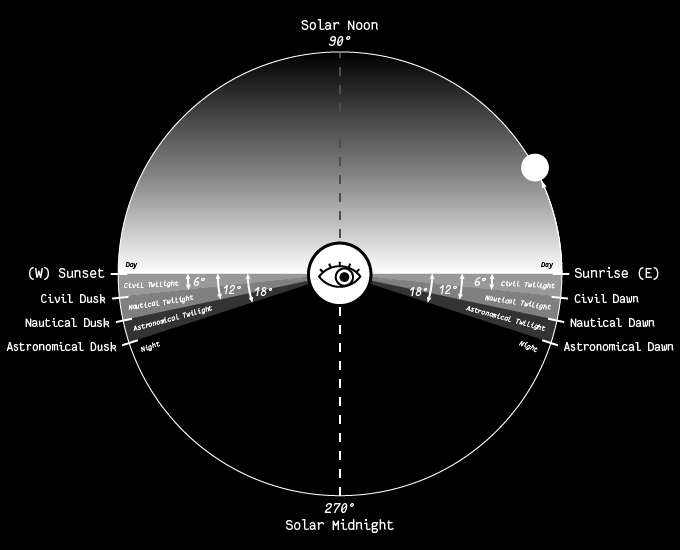## Twilight on Equinox

###### September 22, 2020

Today marks the September Equinox and the beginning of astronomical fall (for the Northern Hemisphere)!

This is one of two times during the year when the Earth’s axis of rotation is parallel to the direction of its orbit around Sun.

This means both hemispheres are equally exposed to the Sun, making day and night nearly equal. At the Equator, the Sun passes directly overhead at noon, and most places on Earth get a similar amount of daylight and darkness.Contrast this with the solstices, when the Earth’s tilt is at its maximum. One hemisphere gets more sunlight over a longer day, marking the height of astronomical summer / winter.A less-intuitive consequence of the solstice-equinox progression (at least for me) is that sunrises, sunsets, twilight, and magic hours are shortest near equinoxes, and longest around the solstices.

To understand why this is true, consider the definition of Twilight. Twilight describes a span of time where the Sun is at a particular altitude, which we measure as a number of degrees above or below the horizon. For example, civil twilight is the period where the Sun is -6 to -12 degrees below the horizon.On equinox, the Sun rises due east, and sets due west. During sunset, this means that the Sun approaches the horizon from directly above. This corresponds to the way we measure altitude, which is perpendicular to the horizon.

On solstice, the Sun sets farther north (or south) of due west, approaching the horizon at a shallower angle. This angled path is longer than the perpendicular one because over the same vertical distance (or altitude) there is more horizontal distance to traverse. The Sun spends more time moving through each degree of altitude, making twilight longer!

Another way to think about it is to consider the circular path the Sun traces around the Earth every day. On equinoxes, this path is the longest of the year (encompassing the entire celestial equator). It takes 24 hours for the Sun to complete this circle, regardless of its size.

From a terrestrial perspective, this means that the Sun has the longest distance to travel through the sky in the same period of time, making its apparent movement “fastest” on equinoxes.

For a deeper dive check out Daniel V. Schroeder’s excellent post on the Sun and Seasons.

— Andrew